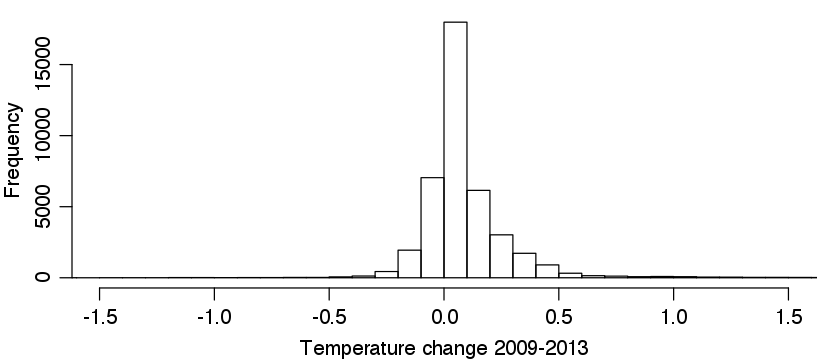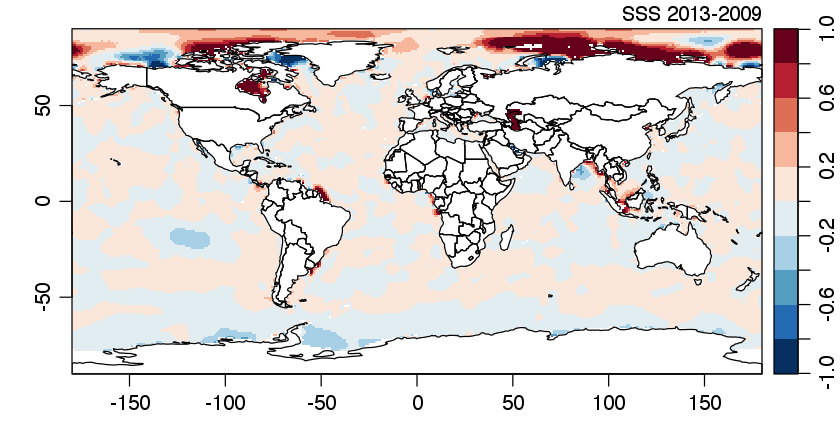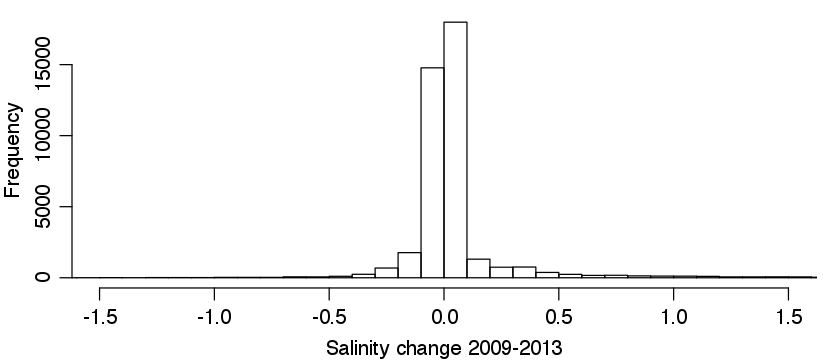Want to share your content on R-bloggers? click here if you have a blog, or here if you don't.

# Introduction

The `ocedata` R package has a dataset named `levitus`, which stores sea-surface temperature and salinity. Today, the development version of `ocedata` (available at github) was updated from the 2009 version of this atlas to the 2013 version. Differences between the two datasets are illustrated here.

# Methods

The code provided below requires that the old dataset be saved into an RDA file. The other trick is that 2009 dataset had longitude running from 0 to 360, whereas the 2013 version has it running from -180 to 180, so the matrices have to be sliced and diced a little bit.

Temperature comparison.

 ``` 1 2 3 4 5 6 7 8 9 10 11 12 13 14 15 16``` ```library(oce) load("~/ocedata-levitus-before-20150126.rda") SST2009 <- levitus\$SST SST2009[1:180,] <- levitus\$SST[181:360,] SST2009[181:360,] <- levitus\$SST[1:180,] data(levitus, package="ocedata") data(coastlineWorld) imagep(levitus\$longitude, levitus\$latitude, levitus\$SST, zlab="SST 2013", col=oceColorsJet, asp=1) lines(coastlineWorld[["longitude"]], coastlineWorld[["latitude"]]) imagep(levitus\$longitude, levitus\$latitude, levitus\$SST-SST2009, zlab="SST 2013-2009", asp=1, breaks=seq(-1,1,0.2)) lines(coastlineWorld[["longitude"]], coastlineWorld[["latitude"]]) par(mar=c(3, 3, 0.5, 1), mgp=c(2, 0.7, 0)) hist(levitus\$SST-SST2009, breaks=100, xlim=c(-1.5, 1.5), xlab="Temperature change 2009-2013", main="") ```

Salinity comparison.

 ``` 1 2 3 4 5 6 7 8 9 10 11 12 13 14 15 16 17``` ```library(oce) load("~/ocedata-levitus-before-20150126.rda") SSS2009 <- levitus\$SSS SSS2009[1:180,] <- levitus\$SSS[181:360,] SSS2009[181:360,] <- levitus\$SSS[1:180,] data(levitus, package="ocedata") data(coastlineWorld) imagep(levitus\$longitude, levitus\$latitude, levitus\$SSS, zlab="SSS 2013", col=oceColorsJet, asp=1) lines(coastlineWorld[["longitude"]], coastlineWorld[["latitude"]]) dev.off() imagep(levitus\$longitude, levitus\$latitude, levitus\$SSS-SSS2009, zlab="SSS 2013-2009", asp=1, breaks=seq(-1,1,0.2)) lines(coastlineWorld[["longitude"]], coastlineWorld[["latitude"]]) par(mar=c(3, 3, 0.5, 1), mgp=c(2, 0.7, 0)) hist(levitus\$SSS-SSS2009, breaks=100, xlim=c(-1.5, 1.5), xlab="Salinity change 2009-2013", main="") ```

# Results

Temperature comparison.Salinity comparison.